# Praxis I Math: Congruence and Similarity Chapter Exam

Exam Instructions:

Choose your answers to the questions and click 'Next' to see the next set of questions. You can skip questions if you would like and come back to them later with the yellow "Go To First Skipped Question" button. When you have completed the practice exam, a green submit button will appear. Click it to see your results. Good luck!

### Page 1

#### Question 2 2. What is the perimeter of the pictured square?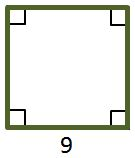#### Question 5 5. If the two triangles pictured below are similar, then what is x?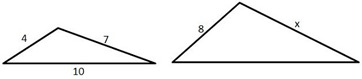### Page 2

#### Question 6 6. Which kind of transformation would change the purple function below into the yellow one?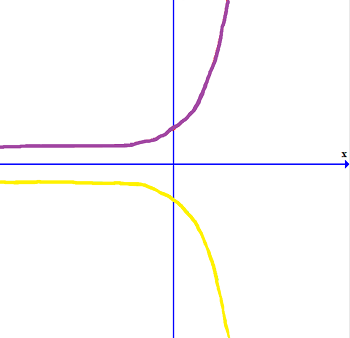#### Question 8 8. If triangle MNO is congruent to triangle PQR, then what is the measure of angle x?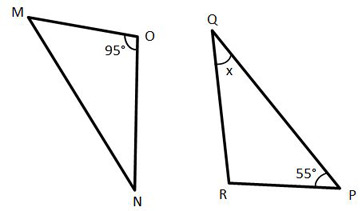#### Question 9 9. The pictured shape is all of the following EXCEPT a: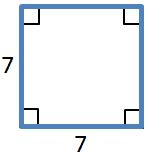#### Question 10 10. The pictured shape is all of the following EXCEPT: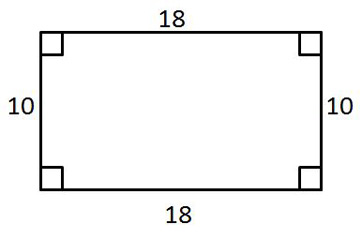### Page 3

#### Question 11 11. If the two triangles pictured below are congruent, then which angles are the same?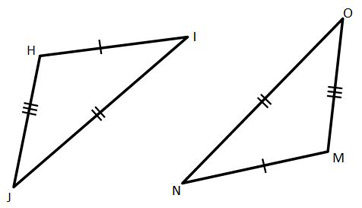#### Question 12 12. Which answer choice is similar to the triangle pictured below?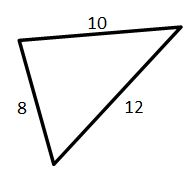#### Question 13 13. What is the scale factor from the smaller rectangle to the larger rectangle pictured below?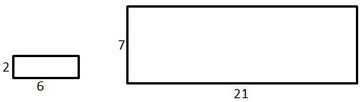#### Question 14 14. What is the area of this trapezoid?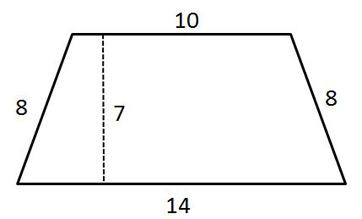#### Question 15 15. Conclude whether triangle BEN is similar to triangle MAT.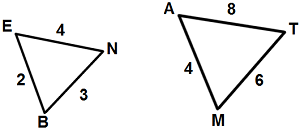### Page 4

#### Question 16 16. Determine if triangle VEZ is similar to triangle MAG.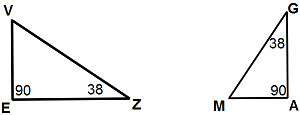#### Question 17 17. Josh claims that these triangles are similar by SSS. Do you agree or disagree with Josh?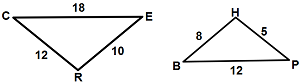#### Question 18 18. In the similar rectangles pictured below, what is y?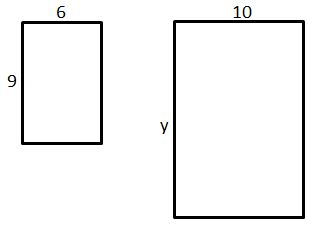#### Question 20 20. Is triangle ABC similar to triangle DEF?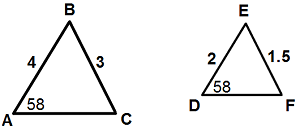### Page 5

#### Question 21 21. What is the area of this parallelogram?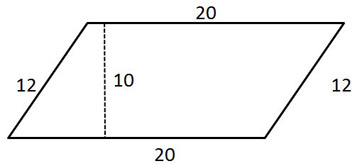#### Question 22 22. In the figure shown below, the graph of the yellow function is a translation of the graph of the blue function. If the expression for the blue function is y(x) = b(x), which of the following is the equation of the yellow function?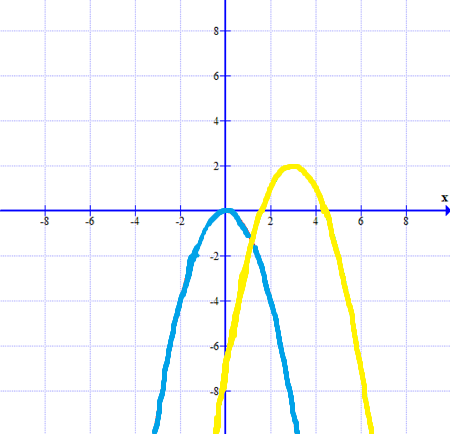#### Question 25 25. What is the perimeter of this polygon?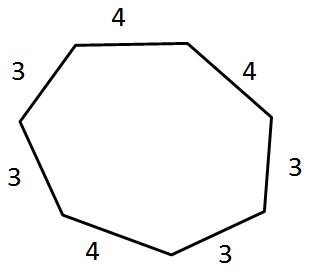### Page 6

#### Question 27 27. The pictured shape is a: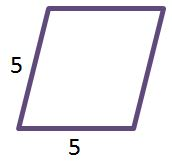#### Question 28 28. If we apply a scale factor of 4 to the triangular prism below, what will the volume of the new prism be?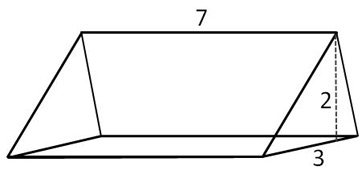#### Question 29 29. Which of the following rectangles represents a scale factor of 3 from the rectangle pictured below?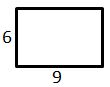#### Question 30 30. Mary claims that the triangles below are similar by AA. Do you agree or disagree with Mary?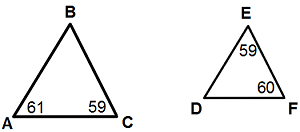#### Praxis I Math: Congruence and Similarity Chapter Exam Instructions

Choose your answers to the questions and click 'Next' to see the next set of questions. You can skip questions if you would like and come back to them later with the yellow "Go To First Skipped Question" button. When you have completed the practice exam, a green submit button will appear. Click it to see your results. Good luck!

Support